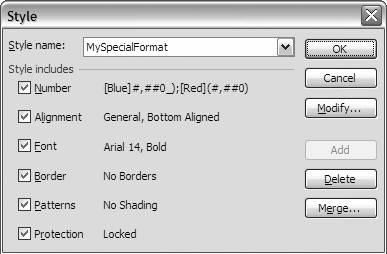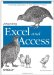# 3.4. Formatting Techniques

 < Day Day Up >

There are essentially two formatting methods, which are on a worksheet using VBA. The first method is to learn where all of the formatting options are and write the code from scratch. This method is most effective when doing the same thing many times (formatting numbers as currency, for example); however, trying to remember how to perform every type of formatting becomes a daunting task. So, this brings us to the second method: using the Macro Recorder .

To access the Macro Recorder from the Excel user interface, go to ToolsMacroRecord New Macro. If all you need is the formatting, pick one cell for each type of formatting that you need to perform. When you are done doing the formatting, press the Stop button to stop recording, or go to ToolsMacroStop Recording. Go to the Visual Basic Editor to view the code that the Excel Macro Recorder wrote to perform the formatting. This gives you the syntax needed for your code. If you always have the same number of rows, etc., you can just do all the formatting and then save the macro to run in the future. If you need to customize it, the next couple of examples show you how.

Determining how to refer to your range becomes tricky. For example, let's assume that you want to format a column as currency (\$0.00). If you are certain that you want every cell in that column to be currency, you can set your range to be that column. In Excel, there is a property called Columns that is available on a Range object and a Worksheet object. If your range is already defined in a variable called xlrng, and you want to perform formatting on the third column, refer to this column as xlrng.columns(3). If you do this, I suggest having an additional range object that you can refer to. If you have a range object called xlrng2, you can write a line that says set xlrng2 = xlrng.columns(3). I like to do this so that the range properties and methods will be available as I write the code.

To explain that a little, if you have a worksheet that is currently active that you refer to as ActiveSheet, you can write ActiveSheet.Range("A1").Value = 100, and it will put 100 in cell A1. However, as you type, VBA will not help you. If you say set xlws = ActiveSheet and type xlws., as soon as you type the . you will see all of the properties and methods available. Using this technique for your objects will make coding much easier. If you have ever tried to script an Excel worksheet in VB script, you can appreciate how useful the help is while typing.

In addition to using the RANGE object, you can also use the Worksheet object to refer to a column. So, if you have a variable xlws that is defined as the ActiveSheet, you can refer to column B by writing xlws.columns(2) or xlws.columns("B:B"). You can also refer to columns B through D by writing xlws.columns("B:D"). If you do not want to refer to entire columns and the range with your data has not been defined yet, you need a method to refer to this set of data. The following example will show you how to select from cell A1 to the end of the worksheet that has data in it.

`     Public Sub SelActiveRange( )     Dim rng As Excel.Range     Dim xlws As Excel.Worksheet     Set xlws = ActiveSheet     Set rng = xlws.Range(xlws.Range("A1"), xlws.Cells.SpecialCells(xlCellTypeLastCell))     rng.Select     Set rng = Nothing     Set xlws = Nothing     End Sub `

The first thing you will notice is that you can refer to a range by using the A1 notation. So, to refer to cell A1, you can write xlws.Range("A1"). You could also do this by writing xlws.cells(1,1) the cells collection also returns a range object that refers to a single cell.

The second method is called SpecialCells. The SpecialCells method returns a range object based on constants built into Excel. I use xlCellTypeLastCell, a special cell that refers to the last cell being used. Of the others you can use, two that I find particularly useful are xlCellTypeFormulas and xlCellTypeComments. These return a range that includes all of the cells that have either formulas or comments, respectively.

Often, I want to send an Excel Worksheet to someone, but I don't want the recipient to change something on one sheet that will affect formula results on another. For example, if you show a scenario of net income based on sales assumptions, and you do not want that net income scenario to be changed if the sales assumptions change, you can change all of the formulas to values using the following procedure. This allows you to maintain a history of the results at various stages. For example, if you make a copy of the scenario with one sales assumption and then remove the formulas, you can maintain the results while still having a second worksheet that will change when the sales assumptions change. Note that the following example assumes that your report is in cells A1 to D100. (You could also build that first range dynamically by using the xlCellTypeLastCell method used above instead of building it directly into the code if you needed more flexibility or if the size of your spreadsheet will grow larger.)

`     Public Sub FormulatoConstant( )     Dim rng As Excel.Range     Dim xlws As Excel.Worksheet     Dim rng2 As Excel.Range     Set xlws = ActiveSheet     Set rng = xlws.Range("A1:D100").SpecialCells(xlCellTypeFormulas)     For Each rng2 In rng.Cells       rng2.Copy       rng2.PasteSpecial xlPasteValues     Next rng2     xlws.Range("A1").Select     Application.CutCopyMode = False     Set rng = Nothing     Set rng2 = Nothing     Set xlws = Nothing     End Sub `

This procedure uses a For Each...Next loop to loop through every cell in the Range variable rng. Each individual cell is copied and then pasted back onto itself with only the values. When it is done, cell A1 is selected and the CutCopyMode is set to False (if you do not set the CutCopyMode to False, cell A1 will be selected, but the last cell copied will still have the marquee box around it as if it is being copied). You could select the entire sheet and use Copy and Paste Special Values, or a For Each...Next loop can be placed into code and set to a particular range by changing it like this:

`     Public Sub FormulatoConstant(targetrange As Excel.Range)     Dim rng As Excel.Range     Dim xlws As Excel.Worksheet     Dim rng2 As Excel.Range     Set xlws = ActiveSheet     Set rng = targetrange.SpecialCells(xlCellTypeFormulas)     For Each rng2 In rng.Cells       rng2.Copy       rng2.PasteSpecial xlPasteValues     Next rng2     xlws.Range("A1").Select     Application.CutCopyMode = False     Set rng = Nothing     Set rng2 = Nothing     Set xlws = Nothing     End Sub `

Using For Each...Next allows you to target just the range of cells that you want to find formulas in and change them to values. You could take this code, put it into any of your Excel workbooks, and with a simple call to the procedure, perform this action.

Since this section is about formatting, let's take a look at how you can apply the currency style to the results of any formula that returns a number. (Remember that formulas can return numbers, Boolean values, text, or errors.) We will modify the procedure in the previous example to use currency.

`     Public Sub FormulatoCurrency(targetrange As Excel.Range)     Dim rng As Excel.Range     Dim xlws As Excel.Worksheet     Dim rng2 As Excel.Range     Set xlws = ActiveSheet     Set rng = targetrange.SpecialCells(xlCellTypeFormulas, xlNumbers)     For Each rng2 In rng.Cells       rng2.Style = "Currency"     Next rng2     xlws.Range("A1").Select     Set rng = Nothing     Set rng2 = Nothing     Set xlws = Nothing     End Sub `

You can see that we've changed the setting of the style. Excel has some predefined styles, which you can refer to more easily than you can write a number format. In addition, you can create a new style and refer to it, as you would one of Excel's predefined formats, to format cells the way you want. Let's assume that column D includes numbers, and you want to create a style that is:

• A number with commas

• A whole number (has no decimal places )

• Blue when positive

• Red when negative

• A bold Arial size 14 font

Formatting that several times in your code or by hand would get repetitive and annoying. Instead, you can create code for a style and apply it from the FormatStyle menu or refer to it in code.

The beauty of creating a style is that you can also modify styles that you've already applied using the FormatStyle screen . So, if you wanted to change every cell that has a particular style, rather than having to find each cell on your worksheet, you could simply modify the style you've applied. The style dialog box is in Figure 3-2. The next example shows you how to create the style. The following example shows you how to apply that style to column D.

##### Figure 3-2. The Excel Style dialog box`     Public Sub CreateStyle( )     ActiveWorkbook.Styles.Add "MySpecialFormat"     With ActiveWorkbook.Styles("MySpecialFormat")         .Font.Bold = True         .NumberFormat = "[Blue]#,##0_);[Red](#,##0)"         .Font.Name = "Arial"         .Font.Size = 14     End With     End Sub     Public Sub ApplyStyle( )     ActiveSheet.Columns("D:D").Style = "MySpecialFormat"     End Sub `

Note that all of the boxes are checked in the style dialog box in Figure 3-2. If, for example, you don't want to apply a style to Protection, uncheck that box. In addition, you could modify the code in the With clause to say .IncludeProtection = False. You can also do that with the other options in the figure.

 < Day Day Up >Integrating Excel and Access
ISBN: 0596009739
EAN: 2147483647
Year: 2005
Pages: 132

Similar book on Amazon